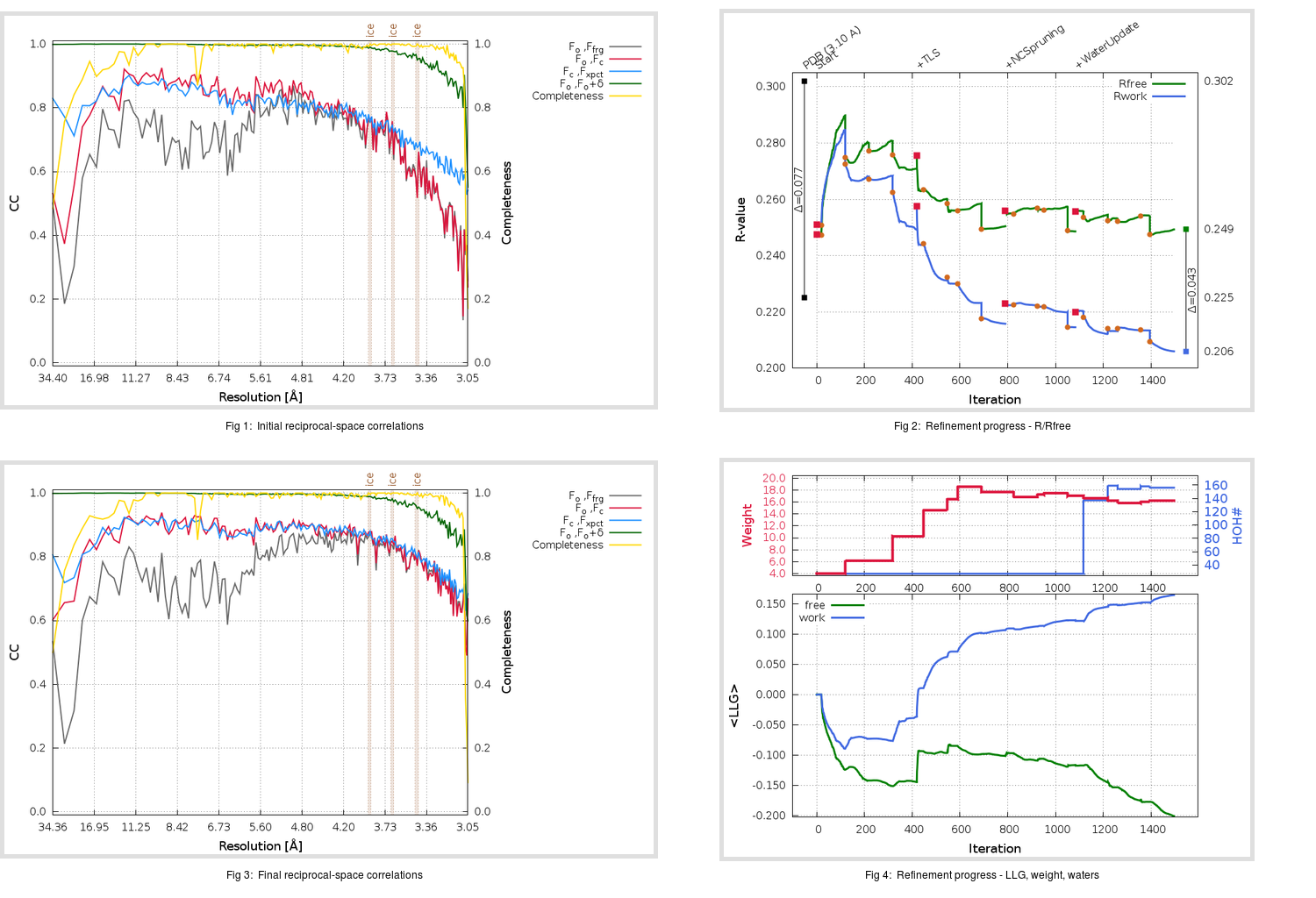Content:

```    Diffraction limits & principal axes of ellipsoid fitted to diffraction cut-off surface:
3.101         0.7234   0.0000   0.6904       0.655 a* + 0.756 c*
3.012         0.0000   1.0000   0.0000       b*
2.985        -0.6904   0.0000   0.7234      -0.540 a* + 0.842 c*
```

## Deposited

` `
 Date deposited Date data collection Resolution R, Rfree 20080501 3.10 0.2210 0.3020

Molprobity (CCP4 7.0 version) summary:

```Ramachandran outliers =   2.88 %
favored =  84.42 %
Rotamer outliers      =   9.96 %
C-beta deviations     =     1
Clashscore            =  18.79
RMS(bonds)            =   0.0105
RMS(angles)           =   1.31
MolProbity score      =   3.20
Resolution            =   3.10
R-work                =   0.2210
R-free                =   0.3020
```

```Number of waters      =    27

<B> (all atoms) =   75.25 ( sd =   22.31 ) for      12643 non-hydrogen atoms
<B>   (protein) =   75.07 ( sd =   22.05 ) for      12522 non-hydrogen atoms
<B>     (water) =   41.69 ( sd =   13.45 ) for         27 non-hydrogen atoms
<B>    (others) =  108.98 ( sd =   25.73 ) for         94 non-hydrogen atoms

B min/max       (all non-hydrogen atoms) =   15.80 /  148.76
B min/max   (protein non-hydrogen atoms) =   26.90 /  144.75
B min/max     (water non-hydrogen atoms) =   15.80 /   71.92
B min/max     (other non-hydrogen atoms) =   52.61 /  148.76
```

## BUSTER (re-)refinement

` `

Molprobity (CCP4 7.0 version) summary:

```Ramachandran outliers =   0.72 %
favored =  93.32 %
Rotamer outliers      =  12.32 %
C-beta deviations     =     8
Clashscore            =   6.74
RMS(bonds)            =   0.0125
RMS(angles)           =   1.62
MolProbity score      =   2.63
Resolution            =   3.05
R-work                =   0.2060
R-free                =   0.2493
```

```Number of waters      =   156

<B> (all atoms) =   93.94 ( sd =   26.81 ) for      12772 non-hydrogen atoms
<B>   (protein) =   93.96 ( sd =   26.11 ) for      12522 non-hydrogen atoms
<B>     (water) =   58.24 ( sd =   13.33 ) for        156 non-hydrogen atoms
<B>    (others) =  149.73 ( sd =   36.63 ) for         94 non-hydrogen atoms

B min/max       (all non-hydrogen atoms) =   31.42 /  201.24
B min/max   (protein non-hydrogen atoms) =   45.96 /  174.90
B min/max     (water non-hydrogen atoms) =   31.42 /  116.39
B min/max     (other non-hydrogen atoms) =   64.76 /  201.24
```

Refinement progression:Results:

` `
 File Remark 3D0H_aB_refine.01_04_refine.pdb.gz exact refinement commands are in header 3D0H_aB_refine.01_04_refine.mtz.gz including original deposited data and several re-refinement map coefficients 3D0H_aB_refine.01_04_BUSTER_model.cif.gz including any non-standard compound restraints 3D0H_aB_refine.01_04_BUSTER_refln.cif.gz